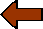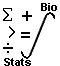## Biostatistics Case Studies## CASE 1: Measures of Central Tendency

### Peripheral Blood Smear:

A WBC count is performed manually by counting 100 WBC's on a smear, typically toward the edge of the smear where the cells are nearly touching but not overlapping. In cases of severe leukopenia it may be possible only to do a 50 cell WBC count.1. Identify the mean, median, and mode in the following series of measurements of hematocrit:

39, 38, 39, 37, 40, 42, 39, 37, 39, 40.

2. A measure of the probability of a distribution of values is known as the central tendency and can be simply calculated as a mean, median, or mode:

• Mean: This is the sum of a list of numbers, divided by the total number of numbers in the list. It is also called the arithmetic mean.

• Median: This is the middle value in a list and is the smallest number such that at least half the numbers in the list are no greater than it. If the list has an odd number of entries, the median is the middle entry in the list after sorting the list into increasing order. If the list has an even number of entries, the median is equal to the sum of the two middle (after sorting) numbers divided by two.

• Mode: For lists, the mode is the most common (frequent) value. A list can have more than one mode.

In the above example, the mean, median, and mode are all 39.